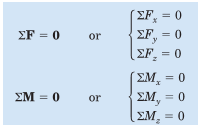Courses

# Condition for Equilibrium in 3D Mechanical Engineering Notes | EduRev

## Mechanical Engineering : Condition for Equilibrium in 3D Mechanical Engineering Notes | EduRev

The document Condition for Equilibrium in 3D Mechanical Engineering Notes | EduRev is a part of the Mechanical Engineering Course Engineering Mechanics - Notes, Videos, MCQs & PPTs.
All you need of Mechanical Engineering at this link: Mechanical Engineering

We now extend our principles and methods developed for two dimensional equilibrium to the case of three-dimensional equilibrium. In Art. 3/1 the general conditions for the equilibrium of a body were stated in Eqs. 3/1, which require that the resultant force and resultant couple on a body in equilibrium be zero. These two vector equations of equilibrium and their scalar components may be written asThe ﬁrst three scalar equations state that there is no resultant force acting on a body in equilibrium in any of the three coordinate directions. The second three scalar equations express the further equilibrium requirement that there be no resultant moment acting on the body about any of the coordinate axes or about axes parallel to the coordinate axes. These six equations are both necessary and sufﬁcient conditions for complete equilibrium. The reference axes may be chosen arbitrarily as a matter of convenience, the only restriction being that a right-handed coordinate system should be chosen when vector notation is used. The six scalar relationships of Eqs. 3/3 are independent conditions because any of them can be valid without the others. For example, for a car which accelerates on a straight and level road in the x-direction, Newton’s second law tells us that the resultant force on the car equals its mass times its acceleration. Thus ΣFx = 0, but the remaining two force–equilibrium equations are satisﬁed because all other acceleration components are zero. Similarly, if the ﬂywheel of the engine of the accelerating car is rotating with increasing angular speed about the x-axis, it is not in rotational equilibrium about this axis. Thus, for the ﬂywheel alone, ΣMx = 0 along with ΣFx = 0, but the remaining four equilibrium equations for the ﬂywheel would be satisﬁed for its mass-center axes. In applying the vector form of Eqs. 3/3, we ﬁrst express each of the forces in terms of the coordinate unit vectors i, j, and k. For the ﬁrst equation, ΣF = 0, the vector sum will be zero only if the coefﬁcients of i, j, and k in the expression are, respectively, zero. These three sums, when each is set equal to zero, yield precisely the three scalar equations of equilibrium, ΣFx = 0, ΣFy = 0, and ΣFz = 0. For the second equation, ΣM

0, where the moment sum may be taken about any convenient point O, we express the moment of each force as the cross product rF, where r is the position vector from O to any point on the line of action of the force F. Thus ΣM = Σ(r*F) = 0.

When the coefﬁcients of i, j, and k in the resulting moment equation are set equal to zero, respectively, we obtain the three scalar moment equations ΣMx = 0, ΣMy = 0, and ΣMz = 0.

Offer running on EduRev: Apply code STAYHOME200 to get INR 200 off on our premium plan EduRev Infinity!

## Engineering Mechanics - Notes, Videos, MCQs & PPTs

30 videos|72 docs|65 tests

,

,

,

,

,

,

,

,

,

,

,

,

,

,

,

,

,

,

,

,

,

;